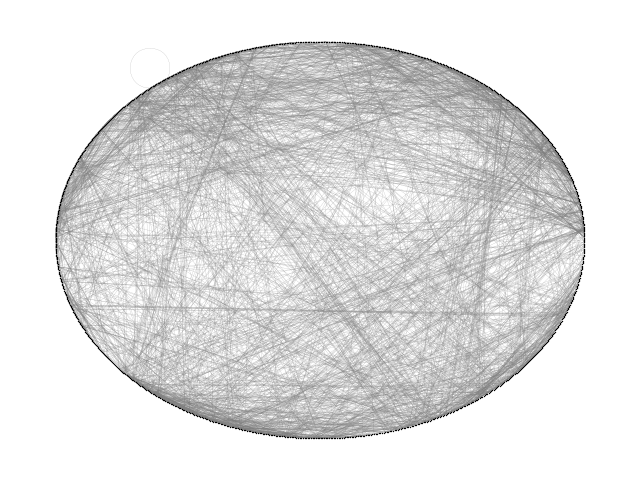# Roget¶

Build a directed graph of 1022 categories and 5075 cross-references as defined in the 1879 version of Roget’s Thesaurus. This example is described in Section 1.2 of

Donald E. Knuth, “The Stanford GraphBase: A Platform for Combinatorial Computing”, ACM Press, New York, 1993. http://www-cs-faculty.stanford.edu/~knuth/sgb.html

Note that one of the 5075 cross references is a self loop yet it is included in the graph built here because the standard networkx `DiGraph` class allows self loops. (cf. 400pungency:400 401 403 405).

The data file can be found at:Out:

```skipping self loop 400 400
DiGraph with 1022 nodes and 5075 edges
21 connected components
```

```import gzip
import re
import sys

import matplotlib.pyplot as plt
import networkx as nx

def roget_graph():
"""Return the thesaurus graph from the roget.dat example in
the Stanford Graph Base.
"""
# open file roget_dat.txt.gz
fh = gzip.open("roget_dat.txt.gz", "r")

G = nx.DiGraph()

line = line.decode()
continue
if line.startswith(" "):  # this is a continuation line, append
line = oldline + line
if line.endswith("\\\n"):  # continuation line, buffer, goto next
oldline = line.strip("\\\n")
continue

numfind = re.compile(r"^\d+")  # re to find the number of this word

for tail in tails.split():
print("skipping self loop", head, tail, file=sys.stderr)

return G

G = roget_graph()
print(G)
UG = G.to_undirected()
print(nx.number_connected_components(UG), "connected components")

options = {
"node_color": "black",
"node_size": 1,
"edge_color": "gray",
"linewidths": 0,
"width": 0.1,
}
nx.draw_circular(UG, **options)
plt.show()
```

Total running time of the script: ( 0 minutes 0.293 seconds)

Gallery generated by Sphinx-Gallery